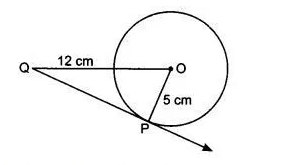# 3.   A tangent PQ at a point P of a circle of radius 5 cm meets a line through the centre O at a point Q so that OQ = 12 cm. Length PQ is :       (A) 12 cm       (B) 13 cm       (C) 8.5 cm       (D) cm.

M manish

The correct option is (d) =  cmIt is given that the radius of the circle is 5 cm. OQ = 12 cm
According to question,
We know that
So, triangle OPQ is a right angle triangle. By using pythagoras theorem,

cm

Exams
Articles
Questions